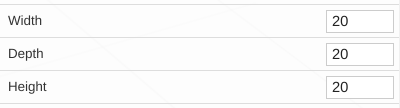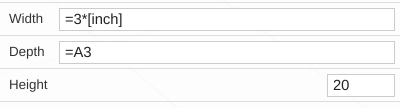The sheet object and system is still very experimental. The data format may change in the future and objects created under the current version may break in the future.

Setting a field to use a function from a sheet

Adding '=' to the beginning of a value allows it to be a an equation or a reference to a sheet (if one is present).

For example:

Here are the settings for a default Cube objectAnd here are the settings that are using a formula and referencing a cell of a tableYou can add constants to your expressions by enclosing them in brackets [].

The Following constants are defined:

Measurement conversions (to milimeters)

• cm - Centimeters = 10
• m - Meters = 1000
• inch - Inch = 25.4
• ft - Feet = 304.8

Standard Math Constants

• pi = 3.14159265359
• tau = 6.28318530718
• e = 2.7182818284
• rand = A random number between 0 and 1

Scripting assistance

• index = The index an object is from an array operation (only working on linear arrays)
• index0 = Same as index (planned)
• index1 = The next (second) array operation up the parent stack (planned)
• index2 = The third array operation up the parent stack (planned)

Creating DesignApps using sheet data

You can add a reference to a sheet cell in the Component Object by adding a line of

![CellID]

for example

!A1

If you would like that edit field to have a friendly name you can rename the cell inside the sheet editor and the cell name will show in the Component.